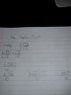# Surds - Division & rationalising

• chuffy

## Homework Statement

simplify 5√20 / 5√15

## The Attempt at a Solution

Looking for someone to check my work

1. multiply (5√20)/(2√15) by (√15/√15)

2. Above gives (5√300)/30

3. Simplify the topline to 5 √100 x √3

I checked my answer using http://www.wolframalpha.com/input/?i=simplify+(5√20)/(2√15)

says I should get 5/√3

thanks

Last edited:
Show how you got from step 2 to step 3 and from step 3 to step 4.

took a pic of my work

#### Attachments

•IMG_20120924_231000.jpg
11.5 KB · Views: 412

## Homework Statement

simplify 5√20 / 5√15

## The Attempt at a Solution

Looking for someone to check my work

1. multiply (5√20)/(2√15) by (√15/√15)

2. Above gives (5√300)/30

3. Simplify the topline to 5 √100 x √3

I checked my answer using http://www.wolframalpha.com/input/?i=simplify+(5√20)/(5√15)
You entered a different problem on wolframalpha. What you entered there was 5√20/(5√15)

The answer you have above is correct but should be reduced.
says I should get 2/√3

thanks

You entered a different problem on wolframalpha. What you entered there was 5√20/(5√15)

The answer you have above is correct but should be reduced.

Yeah looks like I entered that wrong on the website

so (50√3)/ 30 can be tided up by having the √3 on the bottom

gives 150/(30√3)

which gives 5/√3

Yeah looks like I entered that wrong on the website

so (50√3)/ 30 can be tided up by having the √3 on the bottom

gives 150/(30√3)

which gives 5/√3

Usually when you get questions like rationalizing the denominator, it's customary to leave your answer without any surds in the denominator, so even though $5/\sqrt{3}$ looks neater, you should leave your answer as $5\sqrt{3}/3$